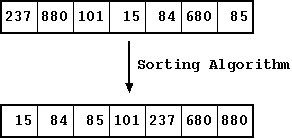## CodingBison

Sorting is one of fundamental concepts that we use every day. Sorting is arranging a list of elements in either increasing or decreasing order of some property. We can arrange them in increasing order or decreasing order. Sorting the employee list based on the property "name" starting from A to Z. When the elements are numericFigure: Algorithm to Sort Elements

We have a wide range of sorting techniques we employee to get the list sorted. We will see some of them in the further sections. Let us define the properties that define the efficiency of an algorithm. First, Time complexity - It is defined as the time taken by the algorithm to finish the execution. Time complexity is usually represented in Big O notation. Second, Space complexity - It is defined as the amount of extra space used in order to finish the execution of algorithm.

Algorithms like merge sort are not in place algorithms, What does this mean ? The extra space used to sort the data set grows with the size of the input data set. On the other hand, Quick sort is an in place algorithm, quick sort rearranges the elements in an array, it uses constant extra space. The extra space used bu the algorithm remains the same irrespective of the input data set size.

#### Sorting Algorithms Types

Based on the implementation we can classify algorithms in to two types. Recursive and non-recursive algorithms. If you are not familiar with recursion, please take a look at the recursion section in our C Module. Quick sort and merge sort are couple of examples of recursive algorithms. Selection sort and Bubble sort are examples of non-recursive Algorithms.

Most of the algorithms (both recursive and no recursive) compare the elements in the list and perform the computations based on the comparison. Hence they are also called comparison algorithms. The best case complexity of a comparison algorithm is O(NlogN). In certain scenarios we can sort the list without comparisons and can achieve the best case complexity of O(N), these techniques are called linear sorting algorithms. Counting sort and Radix sort are examples of linear sorting and we will see more about these techniques in the further sections.

Sorting algorithms can also be classified into two types, internal sorting and external sorting. Internal sorting is something when the data set to be sorted or computations to be done during the sorting process are large enough to be kept in the main memory itself. On the other hand, external sorting is the one where the data set is large and cannot be put in the main memory. It has to deal with copying the chunks of data into the internal memory from the external memory.